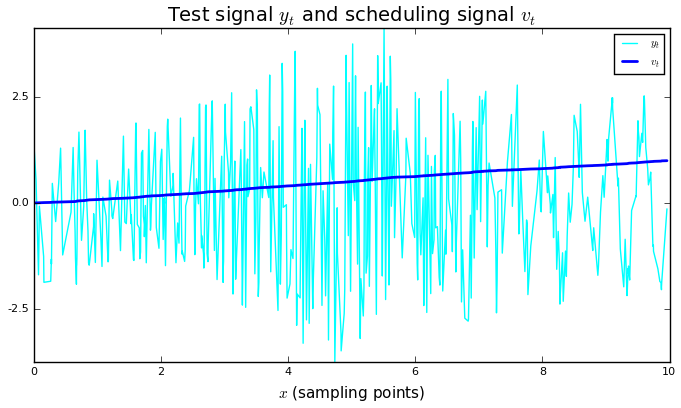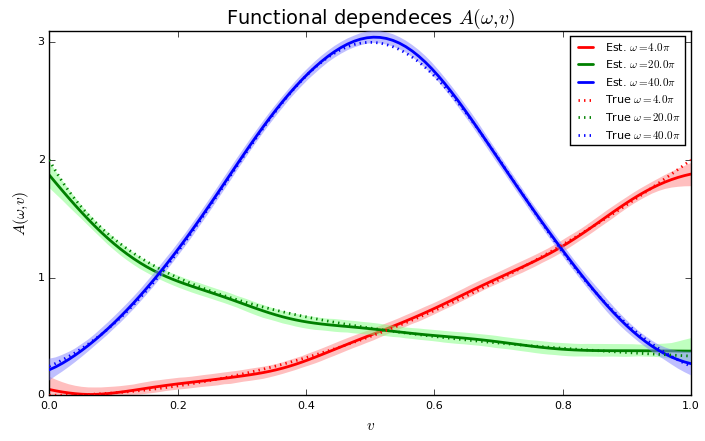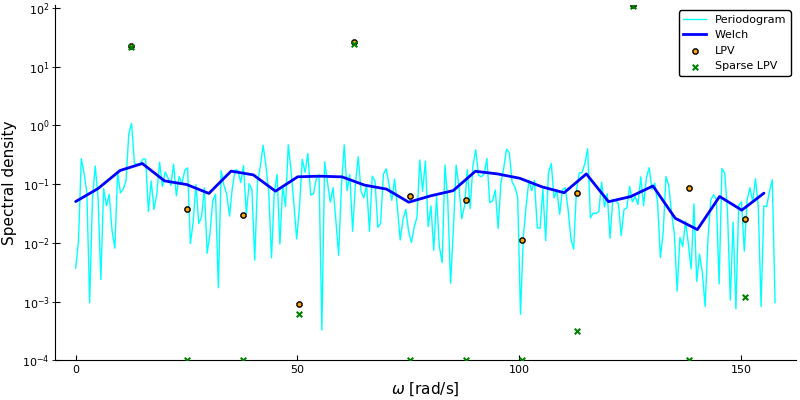6

2

5

7

# LPVSpectral

A toolbox for least-squares spectral estimation, sparse spectral estimation and Linear Parameter-Varying (LPV) spectral estimation. Contains an implementation of the spectral estimation method presented in Bagge Carlson et al. "Linear Parameter-Varying Spectral Decomposition." 2017 American Control Conference.

@inproceedings{bagge2017spectral,
title        = {Linear Parameter-Varying Spectral Decomposition},
author       = {Bagge Carlson, Fredrik and Robertsson, Anders and Johansson, Rolf},
booktitle    = {2017 American Control Conference (ACC)},
year         = {2017},
}


Extensions (sparse estimation methods) to the above article were developed in Bagge Carlson, F., "Machine Learning and System Identification for Estimation in Physical Systems" (PhD Thesis 2018).

@thesis{bagge2018,
title        = {Machine Learning and System Identification for Estimation in Physical Systems},
author       = {Bagge Carlson, Fredrik},
keyword      = {Machine Learning,System Identification,Robotics,Spectral estimation,Calibration,State estimation},
month        = {12},
type         = {PhD Thesis},
number       = {TFRT-1122},
institution  = {Dept. Automatic Control, Lund University, Sweden},
year         = {2018},
url          = {https://lup.lub.lu.se/search/publication/ffb8dc85-ce12-4f75-8f2b-0881e492f6c0},
}


## Installation

import Pkg; Pkg.add("LPVSpectral")

# List of functions

This package provides tools for general least-squares spectral analysis, check out the functions

ls_spectral             # Least-squares spectral analysis
ls_sparse_spectral      # Least-squares sparse (L0) spectral analysis (uses ADMM)
tls_spectral            # Total Least-squares spectral analysis
ls_windowpsd            # Windowed Least-squares spectral analysis (sparse estimates available, see kwarg estimator)
ls_windowcsd            # Windowed Least-squares cross-spectral density estimation (sparse estimates available, see kwarg estimator)
ls_cohere               # Least-squares cross coherence estimation (sparse estimates available, see kwarg estimator)
ls_spectral_lpv         # LPV spectral decomposition
ls_sparse_spectral_lpv  # LPV spectral decomposition with group-lasso penalty on frequencies (uses ADMM)
ls_windowpsd_lpv        # Windowed power spectral density estimation with LPV method

mel                     # Compute Mel projection matrix
melspectrogram          # Standard Mel spectrogram
mfcc                    # Mel cepstrum spectrogram


The functions that estimate sparse spectra require the user to manually import using ProximalOperators.

All functions have docstrings available in the REPL. The general pattern is

x,f = ls_XXX(y,t,f=default_freqs(t) [, W]; kwargs...)


where x are the complex Fourier coefficients and f are the frequency points. If no frequency vector is supplied, the default is to assume a sample time of 1 and use an equidistant grid from 0 to 0.5 of length(t)÷2. W is an optional weight vector of length(y) for weighted least-squares estimation. Some methods accept keyword arguments, these methods are ls_windowpsd, ls_windowcsd, ls_cohere and the keywords and their defaults are nw = 10, noverlap = -1, window_func=rect, estimator=ls_spectral.

# Sparse spectral estimation

We provide a number of ways to estimate spare spectra. These functions require the user to manually load using ProximalOperators.

## L₁ regularized spectral estimation

Minimize ||y-Ax||₂² + λ||x||₁ where x are the Fourier coefficients. Promotes a sparse spectrum

x = ls_sparse_spectral(y,t,ω; proxg=NormL1(λ), tol=1e-9, printerval=1000, iters=30000, μ=0.000001)


## L₀ regularized spectral estimation

Minimize ||y-Ax||₂² + λ||x||₀ where x are the Fourier coefficients. Promotes a sparse spectrum

x = ls_sparse_spectral(y,t,ω; proxg=NormL0(λ), tol=1e-9, printerval=1000, iters=30000, μ=0.000001)


## L₀ constrained spectral estimation

Minimize ||y-Ax||₂² s.t. ||x||₀ ≦ r where x are the Fourier coefficients. Enforces an r-sparse spectrum

x = ls_sparse_spectral(y,t,ω; proxg=IndBallL0(r), tol=1e-9, printerval=1000, iters=30000, μ=0.000001)


## Sparse LPV spectral estimation

See detailed example below and Bagge 2018.

se = ls_sparse_spectral_lpv(Y,X,V,ω_test,Nv; λ = 0.1, normalize = normal, tol=1e-8, printerval=100, iters=6000)


# LPV spectral estimation

We demonstrate the usage of the package with a simple example using simulated data, details can be found in the paper.

## Signal generation

using LPVSpectral, Plots, LaTeXStrings, DSP

"""
y,v,x = generate_signal(f,w,N)

f is a vector of functions f(v) that determine the functional dependence of the spectrum upon the velocity, one function for each frequency in w  both the amplitude and the phase are determined from these functions

w is a vector of frequencies for which to estimate the spectrum

y,v,x are output signal, sample points and scheduling variable respectively
"""
function generate_signal(f,w,N, modphase=false)
x = sort(10rand(N)) # Sample points
v = range(0, stop=1, length=N) # Scheduling variable

# generate output signal
dependence_matrix = Float64[f[(i-1)%length(f)+1](v) for v in v, i in eachindex(w)] # N x nw
frequency_matrix  = [cos(w*x -0.5modphase*(dependence_matrix[i,j])) for (i,x) in enumerate(x), (j,w) in enumerate(w)] # N x nw
y = sum(dependence_matrix.*frequency_matrix,dims=2)[:] # Sum over all frequencies
y += 0.1randn(size(y))
y,v,x,frequency_matrix, dependence_matrix
end

N      = 500 # Number of training data points
f      = [v->2v^2, v->2/(5v+1), v->3exp(-10*(v-0.5)^2),] # Functional dependences on the scheduling variable
w      = 2π.*[2,10,20] # Frequency vector
w_test = 2π.*(2:2:25) # Test Frequency vector, set w_test = w for a nice function visualization

Y,V,X,frequency_matrix, dependence_matrix = generate_signal(f,w,N, true)


## Signal analysis

We now make use of the spectral estimation method presented in the paper:

# Options for spectral estimation
λ      = 0.02 # Regularization parameter
λs     = 1    # Regularization parameter group-lasso
normal = true # Use normalized basis functions
Nv     = 50   # Number of basis functions

se  = ls_spectral_lpv(Y,X,V,w_test,Nv; λ = λ, normalize = normal) # Perform LPV spectral estimation
ses = ls_sparse_spectral_lpv(Y,X,V,w_test,Nv; λ = λs, normalize = normal, tol=1e-8, printerval=100, iters=6000) # Same as above but with a group-lasso penalty on frequencies, promoting a solution with a sparse set of frequencies. Can be used to identify a sparse spectrum, i.e. to find w among w_test.


All that remains now is to visualize the result, along with the result of standard spectral estimation methods.

plot(X,[Y V], linewidth=[1 2], lab=["\$y_t\$" "\$v_t\$"], xlabel=L"$x$ (sampling points)", title=L"Test signal $y_t$ and scheduling signal $v_t$", legend=true, xlims=(0,10), grid=false, c=[:cyan :blue])
plot(se; normalization=:none, dims=2, l=:solid, c = [:red :green :blue], fillalpha=0.5, nMC = 5000, fillcolor=[RGBA(1,.5,.5,.5) RGBA(.5,1,.5,.5) RGBA(.5,.5,1,.5)], linewidth=2, bounds=true, lab=reshape(["Est. \$\\omega =$(round(w/π))\\pi \$" for w in w_test],1,:), phase = false) plot!(V,dependence_matrix, title=L"Functional dependencies$A(\omega,v)$", xlabel=L"$v$", ylabel=L"$A(\omega,v)$", c = [:red :green :blue], l=:dot, linewidth=2,lab=reshape(["True \$\\omega = $(round(w/π))\\pi\$" for w in w],1,:), grid=false)

# Plot regular spectrum
spectrum_lpv   = psd(se) # Calculate power spectral density
spectrum_lpvs  = psd(ses) # Calculate sparse power spectral density
fs             = N/(X[end]-X) # This is the (approximate) sampling freqency of the generated signal
spectrum_per   = DSP.periodogram(Y, fs=fs)
spectrum_welch = DSP.welch_pgram(Y, fs=fs)
plot(2π*collect(spectrum_per.freq), spectrum_per.power, lab="Periodogram", l=:path, m=:none, yscale=:log10, c=:cyan)
plot!(2π*collect(spectrum_welch.freq), spectrum_welch.power, lab="Welch", l=:path, m=:none, yscale=:log10, linewidth=2, c=:blue)
plot!(w_test,spectrum_lpv/fs, xlabel=L"$\omega$ [rad/s]", ylabel="Spectral density", ylims=(-Inf,Inf), grid=false, lab="LPV", l=:scatter, m=:o, yscale=:log10, c=:orange)
plot!(w_test,spectrum_lpvs/fs, lab="Sparse LPV", l=:scatter, m=:o, c=:green)When the three frequencies in w have been identified, w_test can be replaced by w for a nicer plot. As indicated by the last figure, the sparse estimate using group-lasso is better at identifying the three frequency components present (with a small bias in the estimation of the true frequencies).

# Plotting

This package defines a recipe for plotting of periodogram types from DSP.jl. You can thus type

using LPVSpectral, DSP, Plots
plot(periodogram(y))
plot(welch_pgram(y))
plot(melspectrogram(y)) # melspectrogram, mel, mfcc are defined in this package


01/23/2017

5 days ago

149 commits